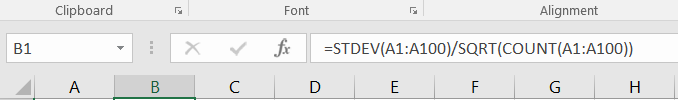# 5. Calculate the standard error of the mean (SEM)

The standard error of the mean may be calculated by dividing the standard deviation by the square root of the number of values in the dataset. There is no direct function in MS Excel to get it automatically. Therefore, you must refer to its definition and type `=STDEV(...)/SQRT(COUNT(...))`.

In our case, simply type `=STDEV(A1:A100)/SQRT(COUNT(A1:A100))`.

NB: since there are two ways to calculate the standard deviation as described here, you may need to adapt the formula above and use either `STDEV.P` or `STDEV.S` instead of `STDEV`. Note that `STDEV` (the function by default) and `STDEV.S` are equal, meaning that `STDEV` assumes that A1:A100 (or any argument placed between parentheses) are a sample of the population, NOT the entire population.

Fant du det du lette etter? Did you find this helpful?
[Average: 5]